803 Completed
18 Videos
8 Exercises
Easy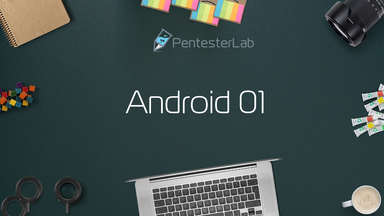Android 01

• This exercise will guide through the process of extracting simple information from an APK
• 1 video
• Completed by 2479 students
• Takes Less than an hour on average
Easy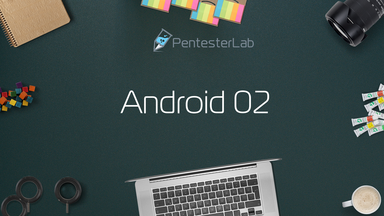Android 02

• This exercise will guide through the process of extracting data from a simple database used by an Android app
• 1 video
• Completed by 2315 students
• Takes Less than an hour on average
Easy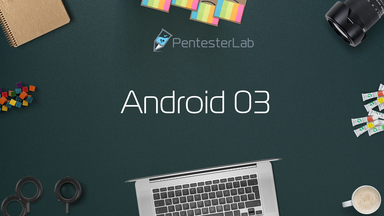Android 03

• This exercise will guide through the process of extracting simple information from an APK
• 1 video
• Completed by 2098 students
• Takes Less than an hour on average
Medium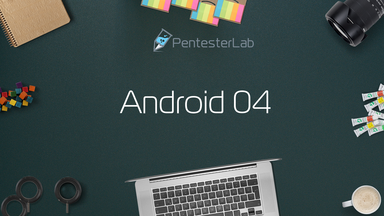Android 04

• This exercise will guide through the process of reversing simple Android code
• 3 videos
• Completed by 1567 students
• Takes Less than an hour on average
Medium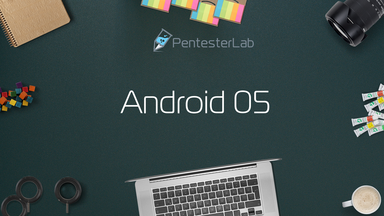Android 05

• This exercise will guide through the process of reversing simple obfuscated Android code
• 3 videos
• Completed by 1224 students
• Takes Between 1 and 2 hours on average
Hard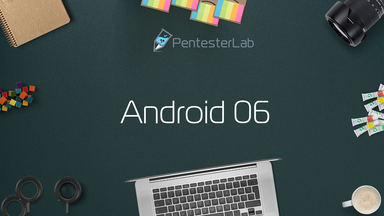Android 06

• This exercise will guide through the process of reversing simple obfuscated Android code
• 3 videos
• Completed by 1019 students
• Takes Between 1 and 2 hours on average
Hard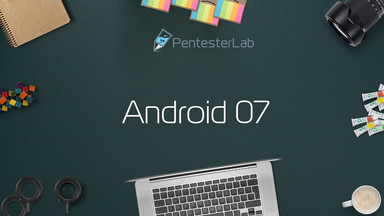Android 07

• This exercise will guide through the process of reversing simple obfuscated Android code
• 3 videos
• Completed by 862 students
• Takes Between 1 and 2 hours on average
Hard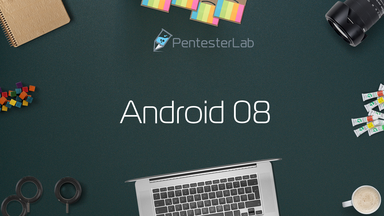Android 08

• This exercise will guide through the process of reversing simple obfuscated Android code to recover encrypted data
• 3 videos
• Completed by 812 students
• Takes Between 1 and 2 hours on average